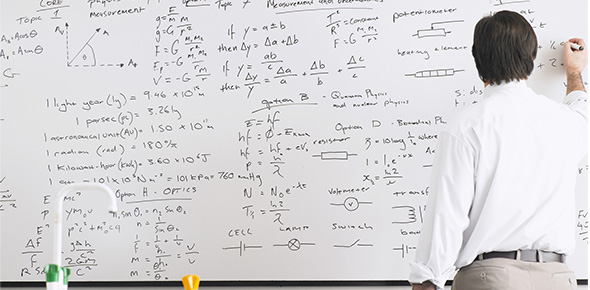# Solving Equations

6 Questions | Total Attempts: 260SettingsThis quiz will help me to know if my students understand how to solve one-step and two-step equations. There will be 6 multiple choice questions.

Related Topics
• 1.
Which equation represents the sentence "The difference of 9 and x is 5."?
• A.

9 = x - 5

• B.

9 = 5 - x

• C.

9 - x = 5

• D.

X - 9 = 5

• 2.
Which equation does not have 6 as a solution?
• A.

R + 5 = 11

• B.

3 - r = -3

• C.

7r = 42

• D.

24/ r = 3

• 3.
What is the solution of y + 31 = 19?
• A.

-50

• B.

-12

• C.

12

• D.

50

• 4.
What is the solution of -20 = g - 4?
• A.

-24

• B.

-16

• C.

5

• D.

80

• 5.
The perimeter of a triangle is 15 units.  The side lengths are (x + 2), (3x - 4), and 5.  What is the value of x?
• A.

1

• B.

2

• C.

3

• D.

4

• 6.
What is the solution of the equation?  -4n - 20 = -32
• A.

3

• B.

13

• C.

-13

• D.

-12